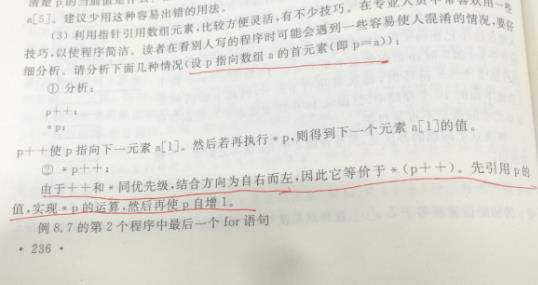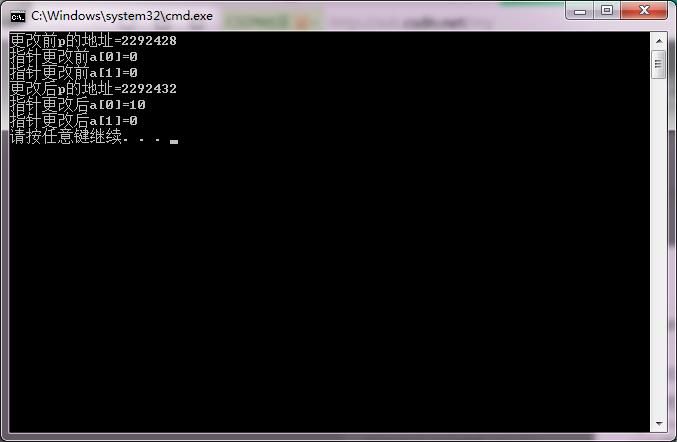C语言指针问题，*p++问题#include
void main()
{
int a={0,0,0,0};
int *p;
p=a;
printf("更改前p的地址=%d\n",p);
printf("指针更改前a=%d\n",a);
printf("指针更改前a=%d\n",a);
*p++=10;
printf("更改后p的地址=%d\n",p);
printf("指针更改后a=%d\n",a);
printf("指针更改后a=%d\n",a);
}4个回答

*p++=10;

p++的意思为：(p++) 指的是指针的移动
(*p)++才是*p=*p+1

p++等于（p++）。接着我们来看这个括号内表达式的值p++。假设表达式的结果为a。那么则为a=p++ ，先进行赋值a=p,然后才是p=p+1。所以: （*（p++））=*（a）即为p自加之前的值。。建议不懂得，在回顾一下自加自减运算c语言链表指针的指针问题

#include<stdio.h> int main() { int a,b; int *p1,*p2; scanf("%d,%d",&a,&b); p1=&a; p2=&b; printf("The number is:%d,%d\n",*p1,*p2); } 输出第二个指针变量所指的值输出总是35是为什么 ![图片说明](https://img-ask.csdn.net/upload/202003/06/1583506967_109380.png)
C语言指针问题，通过指针交换地址

C语言指针问题，*p++问题

c语言结构体成员指针运算的问题

VS2017中C语言指针的问题

c语言指针数组问题，数组内换元素
//输入10个整数 将其中最小的数与第一个数对换，把最大的数与最后一个数对换 #include <stdio.h> void input(int *p); void deal(int *a); void output(int *p); void main() { int a; input(a); deal(a); output(a); } void input(int *p) { int i; printf("请输入十个整数：\n"); for(i=0; i<10; i++) scanf("%d",&p[i]); } void deal(int *a)//这个函数是如何实现第一个数换了之后 第二个数及后面的数自动后退一位呢？ { int *max,*min,*p,temp; max=min=a; for(p=a;p<a+10;p++) if(*p<*min) min=p; temp=a; a=*min; *min=temp; for(p=a;p<a+10;p++) if(*p>*max) max=p; temp=a; a=*max; *max=temp; } /*void deal(int *p) //想问一下这个函数是哪里出错了呢？ { int i,temp1, temp2, t1, t2; temp1=*p; temp2=*p; for(i=1; i<10; i++) { if(temp1 >= *(p+i)) { t1=*(p+i); *(p+i)=temp1; temp1=t1; } } *p=temp1; for(i=1; i<10; i++) { if(temp2 <= *(p+i)) { t2=*(p+i); *(p+i)=temp2; temp2=t2; } } *(p+9)=temp2; } */ void output(int *p) { int i; printf("输出数据如下：\n"); for(i=0; i<10; i++) { printf("%-3d",*(p+i)); } printf("\n"); }
C语言指针问题 为什么P和*P的值一样
# include <stdio.h> #include"malloc.h" int main(void) { // int i; int a={2,1,7,88,99}; int (*p); p = a; printf("%d\n", p); printf("%d", *p); } P和*P值一样
c语言指针问题 数据结构

c语言指针问题，谢谢大家

#include<cstdio> int val; int main(void) { for (int i = 0; i < 100; ++i) val[i] = i; int *p1 = &val, *p2 = &val; printf("%d\n", *p2 - *p1); printf("%d\n",p2-p1); return 0; } 为什么*p2-*p1的值和p2-p1的值一样，p2是p2指针的地址，*p2是指针所指向 地址存储的值，结果怎么会相同呢？
c语言指针结构体问题？
int **p; *p=(*int)malloc(sizeof(int)); 可以这样子用吗？代表什么意思呢？指针的指针？
C语言指针问题,求高手解惑
1.假设有一维int a,a代表数组的地址，&a有什么意义呢， 为什么这里a和&a的值相等呢？ 2.假设有二维数组int a,a和&a也相等吗？ 3.这个是谭浩强C程序设计里的例题： ``` #include<stdio.h> int main() { int a={1,3,5,7}; int (*p); p=&a; //第六行 printf("%d\n",(*p)); return 0; } ``` 书中说第六行只能写成p=&a,而不能是批p=a, 书中说p=a 表示p的值是&a,指向a. p=&a表示p指向一维数组（行），（*p）是p所指向行中序列为3的元素。 但实际上我用VC6.0调试时两个都能得到7的答案，只不过当p=&a时，没有报警， 而当p=a时，编译器有预警信息： warning C4047: '=' : 'int (*)' differs in levels of indirection from 'int *' 难道谭浩强的这本书是按旧的C标准写的吗？还是说编译器允许这样的写法？ 4.a和&a无条件等价吗？
c语言指针直接赋值问题

C语言关于指针数组和数组指针
C语言关于指针数组输出的问题 ``` #include "stdio.h" int main() { char *p1 = { 'A','B','C','D' }; char(*p2) = &p1; for (int i = 0; i < 4; i++) { printf("%c\n", *(*(p2 + i))); } system("pause"); return 0; } ``` 这里是输出格式是\* (\*(p2 + i))) 如果替换为\*(\*p2 + i))，则错误！ ``` #include "stdio.h" int main() { char *p1 = { 'A','B','C','D' }; char(*p2) = &p1; for (int i = 0; i < 4; i++) { printf("%c\n", *(*(p2 + i))); } int *p1 = { 9,8,7,6 }; int(*p2) = &p1; for (int i = 0; i < 4; i++) { printf("%d\n", *(*p2 + i)); } system("pause"); return 0; } ``` 这里是输出是\*(\*p2 + i))， 如果替换为\* (\*(p2 + i)))测错误！ **请问如何解释这种情况。思考了很久也不知道怎么理解**
c语言中指针p 和 p++的问题
int main() { int * ptr=(int*)malloc(10);//arr; printf("%d\n",ptr); printf("%d\n",ptr++); } 为什么输出的值是一样的呢？

20道你必须要背会的微服务面试题，面试一定会被问到

Windows可谓是大多数人的生产力工具，集娱乐办公于一体，虽然在程序员这个群体中都说苹果是信仰，但是大部分不都是从Windows过来的，而且现在依然有很多的程序员用Windows。 所以，今天我就把我私藏的Windows必装的软件分享给大家，如果有一个你没有用过甚至没有听过，那你就赚了????，这可都是提升你幸福感的高效率生产力工具哦！ 走起！???? NO、1 ScreenToGif 屏幕，摄像头和白板...

2020 年，大火的 Python 和 JavaScript 是否会被取而代之？
Python 和 JavaScript 是目前最火的两大编程语言，但是2020 年，什么编程语言将会取而代之呢？ 作者 |Richard Kenneth Eng 译者 |明明如月，责编 | 郭芮 出品 | CSDN（ID：CSDNnews） 以下为译文： Python 和 JavaScript 是目前最火的两大编程语言。然而，他们不可能永远屹立不倒。最终，必将像其他编程语言一...

AI 没让人类失业，搞 AI 的人先失业了

《java面试宝典》三 类初始化和类实例化顺序

2020年刚刚开始，就意味着离职潮高峰的到来，我身边就有不少人拿着年终奖离职了，而最让我感到意外的，是一位工作十年的数据分析师也离职了，不同于别人的主动辞职，他是被公司炒掉的。 很多人都说数据分析是个好饭碗，工作不累薪资高、入门简单又好学。然而今年34的他，却真正尝到了中年危机的滋味，平时也有不少人都会私信问我： 数据分析师也有中年危机吗？跟程序员一样是吃青春饭的吗？该怎么保证自己不被公司淘汰...

B站是个宝，谁用谁知道???? 作为一名大学生，你必须掌握的一项能力就是自学能力，很多看起来很牛X的人，你可以了解下，人家私底下一定是花大量的时间自学的，你可能会说，我也想学习啊，可是嘞，该学习啥嘞，不怕告诉你，互联网时代，最不缺的就是学习资源，最宝贵的是啥？ 你可能会说是时间，不，不是时间，而是你的注意力，懂了吧！ 那么，你说学习资源多，我咋不知道，那今天我就告诉你一个你必须知道的学习的地方，人称...

loonggg读完需要2分钟速读仅需 1 分钟大家我，我是你们的校长。前几天，搜狐的董事局主席兼 CEO 张朝阳和搜狐都上热搜了。原因很简单，就是搜狐出了“考勤新规”。一封搜狐对员工发布...

【蘑菇街技术部年会】程序员与女神共舞，鼻血再次没止住。（文末内推）